# AP Calculus BC Practice Test 12

### Test Information9 questions18 minutes

Calculator Disallowed

1. If the MacLaurin series for ex is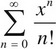, what is a power series expansion for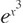?

2. The rate of growth of algae, A, in a pond with respect to time, t, is directly proportional to one half the amount of algae in the pond. Which differential equation describes this relationship?

3. If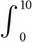f(x) dx = 100 and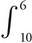f(x) dx = 75, find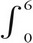f(x) dx.

4.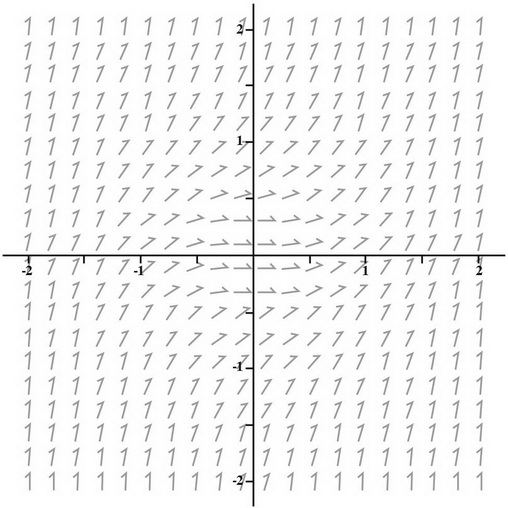Which of the following is the differential equation of the slope field above?

5. The length of a curve from x = 2 to x = 3 is given by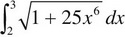. If the curve contains the point (2, 10), which of the following could be the curve?

6. If the line tangent to the graph of the function f at the point (8, 2) passes through the point (2, 5), then f′(8) =

7. A curve is defined by the parametric equations x = t3 + 2t - 1 and y = t2. Which of the following is an equation of the line tangent to the graph at (11, 4)?

8. The graph of the function f is shown below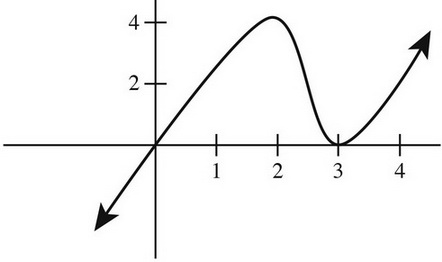If g(x) =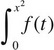dt, what is g′(2)?

9. If the graph of y = f(x) has slope 3x2 + 5 at each point (x,y) on the curve and it passes through (2, 18), which of the following is the equation of f(x)?